Web-Schrödinger 3.3
(C)2007-2021 G. I. Márk, Ph. Lambin, L. P. Biró, EK MFA Budapest,Hungary -- Uni Namur, Belgium
www.nanotechnology.hu

Subscribe to the mailing list to receive E-mail news about Web-Schrödinger (new versions. etc)

Watch introductory videos from the Web-Schrödinger YT channel.

# Introduction

Web-Schrödinger is a program for the interactive solution of the stationary (time independent) and time dependent two dimensional (2D) Schrödinger equation. The program itself runs on our server and can be used through the Internet with a simple Web browser (Internet Explorer, Mozilla, Opera, Chrome was tested). Nothing is installed on the user's computer. The user can load, run, and modify ready-made example files, or prepare her/his own configuration(s), which can be saved on her/his own computer for later use.

See  for a detailed description of the program.

# Theoretical background

## Time dependent Schrödinger equation

The time evolution of the quantum mechanical wave function ψ(r;t) is governed by the time dependent Schrödinger equation: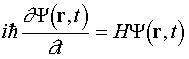where r  = (x,y) is the position coordinate, t is the time and H = K + V is the Hamilton operator, K is the operator of the kinetic energy, and V = V(x,y) is the operator of the potential energy.

When the potential function V(x,y) and the initial wave function ψ(x,y,t0) = ψ0(x,y) is known, the time dependent Schrödinger equation determines the wave function ψ(x,y,t) for any time value. We can calculate all observables from the wave function, for example the rho(x,y,t) probability density and the j(x,y,t) probability current density.

## Stationary Schrödinger equation

rho(x,y,t) gives the probability of finding the quantum mechanical particle around the point (x,y) at time t. We call those ψ(x,y,t)=ψ(x,y) states, where ψ(x,y) is independent of time, stationary states. The stationary (time independent) states are given by the stationary Schrödinger equation:

Hψ(r) = Eψ(r)

where E is the energy of the state.

# User Guide

All functions of the program are available through a menu system. Upon starting the program a default configuration is loaded, the user can immediatelly run this through the Calculation menu, or load another configuration with the Load Example, or Load menu points. All parameters can be modified in the Edit menu and the current setup can be saved anytime with the help of the Save function.

### Files

We have prepared several characteristic examples, illustrating the most important phenomena of quantum mechanics, including the spreading of the wave packet, tunneling, bound states, etc. The current list of the examples is given in Appendix A. The example library is continuously expanding, see Appendix A for the up to date status. After loading an example setup the user can study and modify the parameters through the Edit menu or go straight to Calculation to calculate the time development and/or the stationary states.

This function makes it possible to load the user's own configuration files, from her/his own computer. Such parameter files can be created either by saving a (possibly modified) example configuration (or the default configuration) or writing a configuration file from the scratch with a text editor or any other program.

#### Save

The current state of the parameters can be saved anytime to the user's own computer.

### Edit

#### Mesh

The wave function and the potential is represented on a 2D mesh. Here you can specify the number of mesh points (Nx , Ny) in the x and y direction and the size of the calculation region in Angström (sx, sy). For typical applications the Δx = sx/Nx, Δy = sy/Ny values should be between 0.1 - 1 Å. The origin of the coordinate system is in the middle of the calculation region.

The numerical algorith uses a periodic boundary condition, i.e. what goes out of the calculation region at the right side, comes in at the left side. It is like if the whole plane were "tiled" with the calculation region. As a consequence when the wave packet approaches the boundary of the calculation box, it "meets" its copy at the neighboring box and this causes unphysical interference effects to appear in the probability density. The parameters of the calculation (spatial- and temporal mesh, potential, and initial state) should be carefully chosen to avoid this effect.

V0 gives the default value of the potential in eV (Electronvolt).

Note: due to the difference of the algorithms used for the solution of the time dependent and stationary Schrödinger equations, generally a finer mesh is necessary for the time dependent calculation. E.g. a Nx=256 is typical value for the time dependent, and Nx=64 for the stationary calculation

#### Potential

The potential V(x,y) can be interactively assembled from objects of several types: circle, rectangle, and plane. Any number of these objects can be given. For each object the user can specify its geometrical parameters and its potential value. For pixels where several objects overlap, the object given most recently determines the pixel potential value. The program shows the potential function generated from the current set of objects as a grayscale image.

• The potential for the circle is defined by:
V(r) = V0 + V1 r + V2 r2
• The potential for the plane is defined by:
V(d) = V0 + V1 d + V2 d2,
where d is the (perpendicular) distance from the boundary line
• The potential for the rectangle is defined by:
V(x,y) = V0 + Vx x + Vy y + Vxx x2 + Vyy y2 + Vxy x y ,
where x and y are the (perpendicular)
distances from the x and y sides of the rectangle

#### Initial state

Here the user can specify the initial wave function ψ0(x,y), which is the input of the time dependent calculation (it is not used at the stationary calculation). Its general form is a so called truncated plane wave  wave packet, i.e. a Gaussian wave packet convolved with a 2D square window function. The program displays the chosen initial state together with the potential function, as a composite color image. In order to ensure that the wave packet has its ideal form (minimal size and flat envelope) when it hits the potential, a time retardation procedure is included into the initial state preparation. The user can specify the retardation time by giving the the bx, by distance values, which mean that after proceeding such distances in x, and y the wave packet should have its "ideal" form.

ax, ay give the spatial width of the wave packet. The initial state should be specified such a way, that its overlap with the potential objects is negligible.

#### Detectors

The user can place horizontal or vertical line segments (detectors) into the calculation window. The program calculates the probability current I(t) passing through each line segment during the time evolution of the wave packet and also its time integral T for the whole calculation time. T is called transmission, because it gives the probability that the quantum particle crosses the given line segment (detector).

#### Calculation parameters

Here we can specify the parameters of the time dependent and the stationary calculation.

Parameters used for the time evolution calculation: The number of time points is Nt and Δt gives calculation time step. Δt has to be given in atomic time units, 1 au time = 0.0242 fs (femtosecond).

The numerical algorithm imposes a condition on the maximal Δt value that can be used: Δt < 4/π (Δx)2 / D, where D is the number of dimensions, D=2 in 2D. (This formula is valid in atomic units, i.e. one has to insert Δx in Bohr, 1 Bohr = 0.529 Å. For the default Δx = 0.3 Å, Δt = 0.2 au is suitable and this is the default time step.)

It is not necessary, however, to display the results in such a fine time scale. Therefore the user can input the "display timestep", i.e. the number of calculation time steps, when the wave function is displayed.

Parameters used for the stationary calculation: Nstat gives the number of states calculated.

### Calculation

#### Time development

When the user hits the "RUN" button, the time development calculation starts on the server. The progress of the calculation is shown by small thumbnail images. For typical parameters the time development calculation takes 1-2 minutes. (If there are more concurrent jobs on the server – either from this user or from others – the calculation may be somewhat slower. The program writes out the number of concurrent jobs – if there is any – after hitting the "RUN" button.)

#### Eigenstates

When the user hits the "RUN" button, the calculation of the stationary states starts on the server. It takes several second, or minutes, depending on the mesh size, and the number of orbitals requested. (If there are more concurrent jobs on the server – either from this user or from others – the calculation may be somewhat slower. The program writes out the number of concurrent jobs – if there is any – after hitting the "RUN" button.) When the calculation is completed, the program displays the energies and the wave functions of the stationary states.

### Results

After the time development calculation is completed on the server, the time development of the probability density is displayed in composite color images. The program first calculates the global maximum of the probability and normalizes each frame using this value. A nonlinear color scale (γ=2.5) is used in order to facilitate presentation.

If the user placed detectors into the calculation window before the start of the calculation, the program also displays the I(t) probability current functions and T transmission values for each of the detectors.

# Appendix A: Examples

The examples are diveded into two groups: examples for time development calculation and examples for stationary states calculation. Nothing prevents to perform both a time evolution and a stationary states calculation for the same example, but those examples listed under "time development" demonstrate interesting cases of time development, those listed under "stationary states" demonstrate interesting cases of eiegenstates. For some cases, however, e.g. for a potential box, both the time evolution and the stationary states gives instructive results.

The examples were carefully designed to prevent the effect of the periodic boundary condition. For the time evolution examples, this was accomplished by halting the time development calculation before the wave packet reaches the edge of the calculation box. For the stationary states calculation, we applied a potential wall at the edges in each examples.

## Examples for time development calculation

### band_1D_allowedA wave packet is approaching a periodic potential with energy in the allowed band. The wave packet is passing through the potential.

### band_1D_forbidden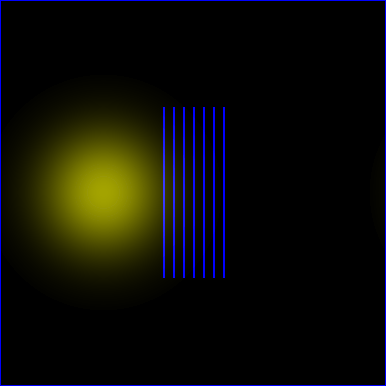A wave packet is approaching a periodic potential with energy in the forbidden band. The wave packet is reflected from the potential.

### ChristmasWave packet scattering on a potential forming a Christmas tree

### gravity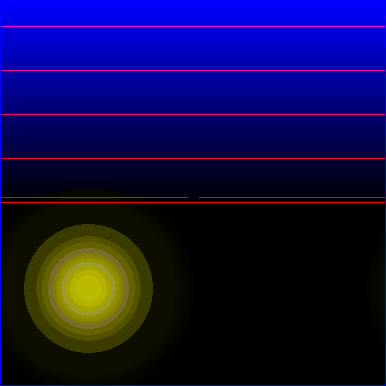Quantum analogue of a projectile motion. Wave packet scattering on a linearly increasing potential. The "Results" menu shows the transferred probabilities and probability densities crossing the detectors shown by the red line segments.

### hardcore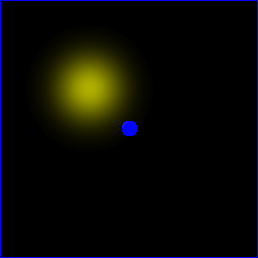Scattering of a wave packet on a circular hardcore potential. Note the circular component of the final state.

### quantum_revival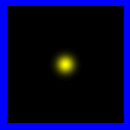Demonstration of the "quantum revival" phenomenon.

### stm_on_nanotube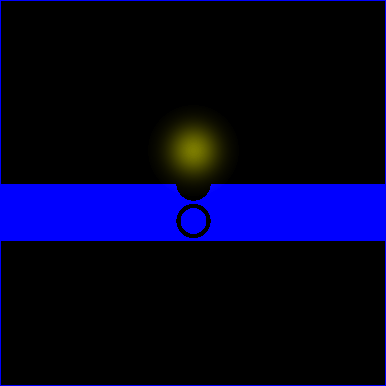Simulation of Scanning Tunneling Microscope imaging of a carbon nanotube. See  for details..

### tunneling_oblique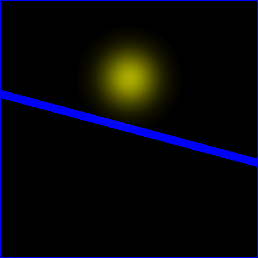Tunneling of a wave packet through a potential wall of V>E. The WP is hitting the wall at 75o angle.

### tunneling_perpendicular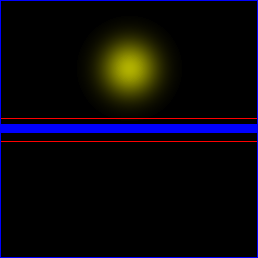Tunneling of a wave packet through a potential wall of V>E. The WP is hitting the wall az 90o angle.

### two_balls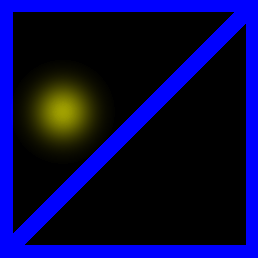Two colliding billiard balls on a 1D track, shown in 2D configuration space. For more explanation, see this video. Comparison of an experiment, a classial mechanics- and quantum mechanics simulation of two colliding billiard balls on a one-dimensional track. Introduction of the concept of configuration space. Comparison of two-particle states for interacting- and non-interacting particles. Two-particle states for interacting particles show Wigner-crystal-like behavior.

### two_pendulums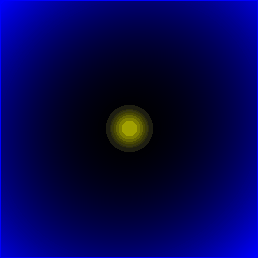Two coupled pendulums, shown in 2D configuration space.

## Examples for stationary states calculation

### boxEigenstates of a rectangular potential box.

### circle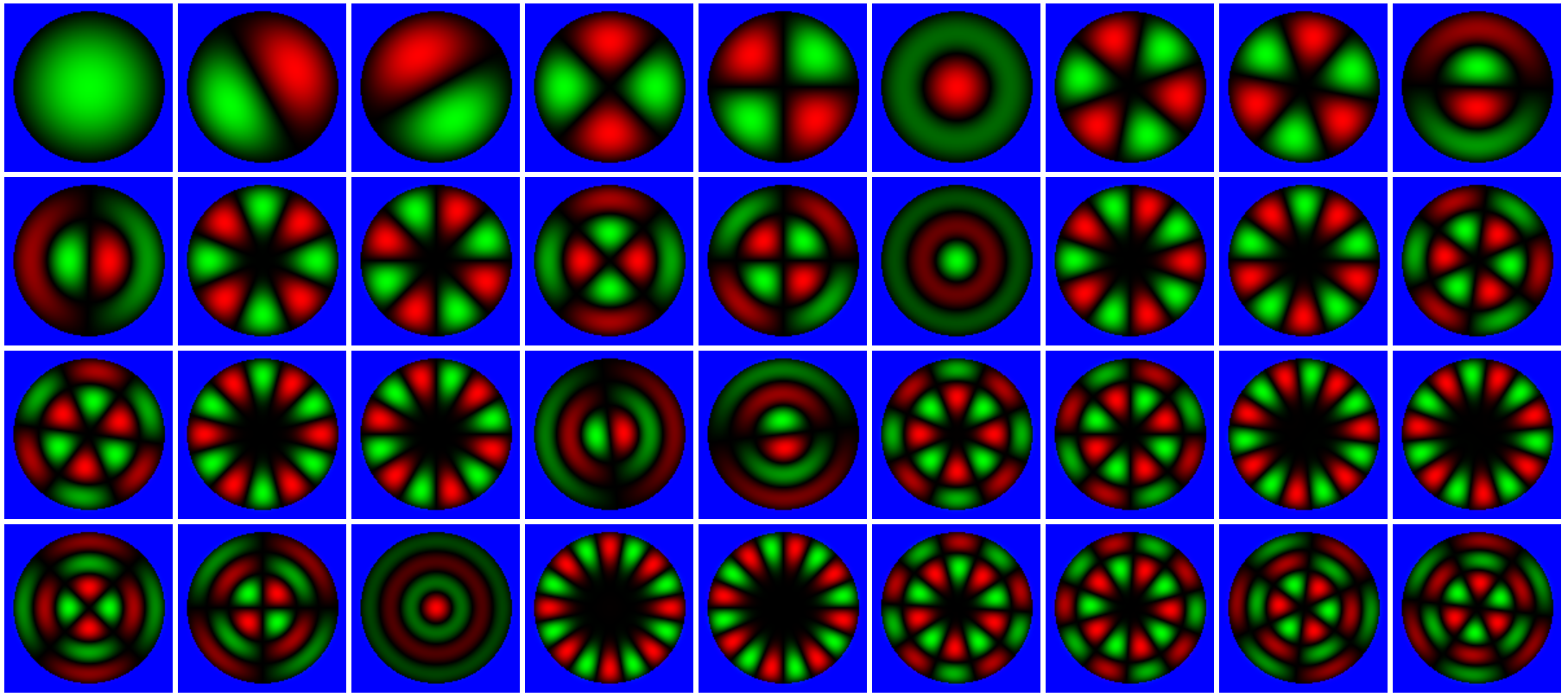Eigenstates of a circular potential box.

### harmosc_2d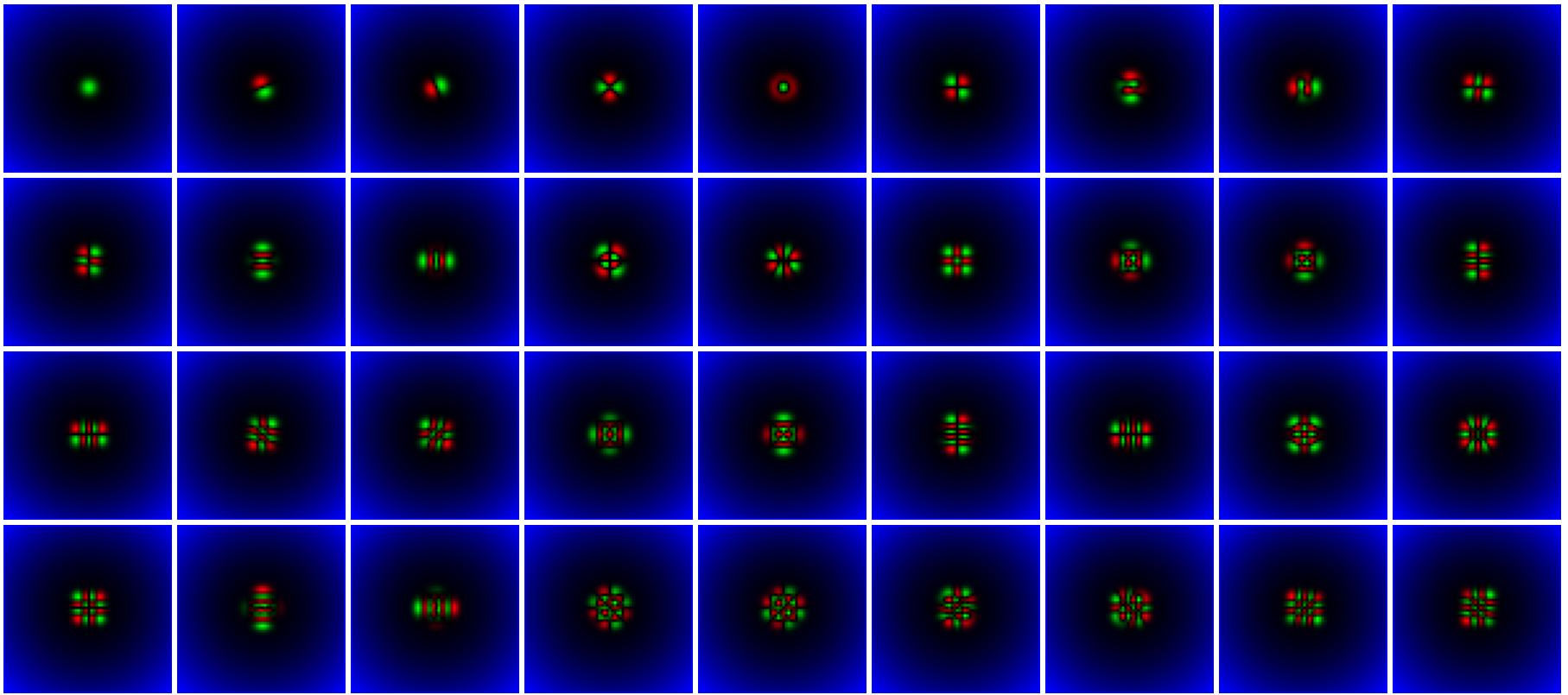### molecule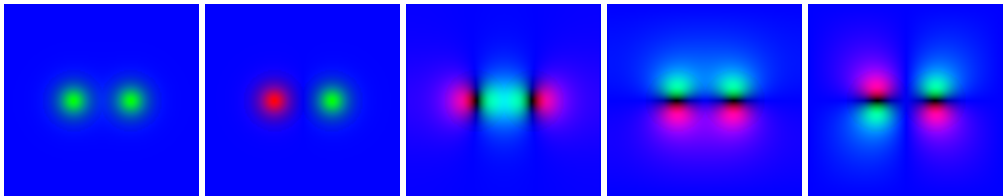Eigenstates of a simple model for a diatomic molecule. Note the two lowest orbitals are "s" like orbitals, similar to the atomic orbitals, the third orbital is a "sigma" orbital, and the fourth and fifth orbitals are "pi" orbitals.

### step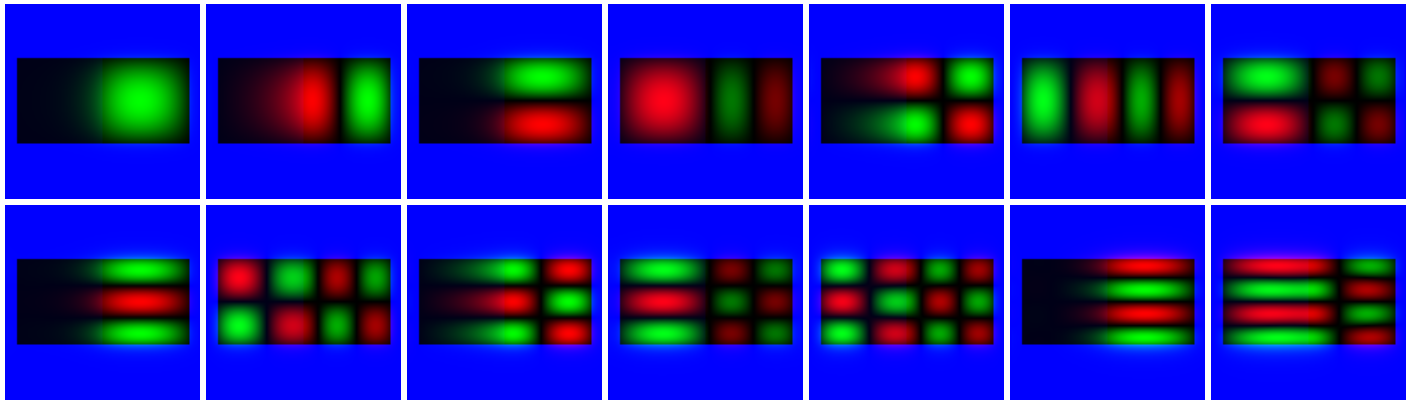This includes a potential step inside a potential box: the left half of the potential has a slightly higher potential value than the righ half.

# Example file contest

Develop your own example files demonstrating interesting quantum phenomena! You can send the SAVE-d files to mark@mfa.kfki.hu . Best example files will be included into the Web-Schrödinger "Examples" directory. Please attach also a brief description of the example!

# Mailing list

We have a mailing list for announcing new features and examples. The mailing list is hosted by Google Groups.

# References

1. Márk, Géza, I.: Web-Schrödinger: Program for the interactive solution of the time dependent and stationary two dimensional (2D) Schrödinger equation;
arXiv:2004.10046 [physics.ed-ph] (2020)
https://arxiv.org/abs/2004.10046

2. Schrödinger equation; (in several languages)
http://en.wikipedia.org/wiki/Schroedinger_equation

3. Time development of quantum mechanical systems; (1995-) (English and Hungarian)
http://www.kfki.hu/~mark/physedu/schrodinger/index.html

4. Márk, Géza, I.; Biró, László, P.; Gyulai, József: Simulation of STM images of 3D surfaces and comparison with experimental data: carbon nanotubes;
Phys. Rev. B 58, 12645(1998).
http://www.nanotechnology.hu/reprint/prb_58_12645.pdf

5. Márk, Géza, I.; Biró, László, P.; Gyulai, József; Thiry, Paul, A.; Lucas, Amand, A.; Lambin, Philippe: Simulation of scanning tunneling spectroscopy of supported carbon nanotubes;
Phys. Rev. B 62, 2797(2000).
http://www.nanotechnology.hu/reprint/prb_62_2797.pdf

6. Lambin, Philippe; Márk, Géza, I.; Meunier, Vincent; Biró, László, P.: Computation of STM images of carbon nanotubes;
Int. J. Qunatum.. Chem. 95, 495(2003).
http://www.nanotechnology.hu/reprint/IntJQuantChem_95_493_STMSimul.pdf

7. Márk, Géza, I.; Biró, László, P.; Lambin, Philippe: Calculation of axial charge spreading in carbon nanotubes and nanotube Y-junctions during STM measurement;
Phys. Rev. B 70, 115423-1(2004).
http://www.nanotechnology.hu/reprint/prb_70_115423_3d.pdf

8. Géza I. Márk PhD Thesis, FUNDP Namur, 2006.
http://www.mfa.kfki.hu/~mark/phd/index.html

9. Márk, Géza, I.; Vancsó, Péter; Hwang, Chanyong; Lambin, Philippe; Biró, László, P.: Anisotropic dynamics of charge carriers in graphene;
Phys. Rev. B 85, 125443-1(2012).
http://www.nanotechnology.hu/reprint/prb_85_125443_graphene_anisotropy_2012.pdf

10. Vancsó, Péter; Márk, Géza, István; Hwang, Chanyong; Lambin, Philippe; Biró, László, P.: Time and energy dependent dynamics of the STM tip – graphene system;
European Journal of Physics B 85, 142-1(2012)
http://www.nanotechnology.hu/reprint/epjb_85_142_graphene_jellium_2012.pdf

11. Márk, Géza, I.; Vancsó, Péter; Lambin, Philippe; Hwang, Chanyong; Biró, László, P.: Forming electronic waveguides from graphene grain boundaries;
Journal of Nanophotonics 6, 061719-1(2012)
http://www.nanotechnology.hu/reprint/jnanophot_6_061718_waveguide2012.pdf

12. S. Janecek, E. Krotscheck: A fast and simple program for solving local Schrödinger equations in two and three dimensions;
Comput. Phys. Comm. 178 (11) (2008) 835–842.
http://www.sciencedirect.com/science/article/pii/S0010465508000453

13. S.A. Chin, S. Janecek, and E. Krotscheck: An arbitrary order diffusion algorithm for solving Schrödinger equations;
Computer Physics Communications 180 (2009) 1700–1708.
http://www.sciencedirect.com/science/article/pii/S0010465509001131

Last updated: February 4, 2021 by Géza I. Márk , mark@mfa.kfki.hu
This page was accessed
times since Feb 8, 2013.